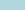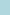### Algebra 1 Worksheets### Algebra 2 Worksheets### Pre-Algebra Worksheets### Elementary Algebra### Algebra Word Problems### Intermediate Algebra### Algebra Calculator### Algebra Worksheets# Algebra Calculator

Use an online algebra calculator to solve equations and get the answers you need. Need algebra help? The algebra calculator is the first place you should start. These free online calculators will be a great resource in your studies. A calculator is a very important device used in mathematics. Students start using them when doing simply arithmetic. Now take the next step and start using algebra calculators when doing your elementary algebra. pre-algebra, algebra 1, and algebra 2 homework. These algebra calculators actually wear several hats. These each can be used as your algebra factoring calculator, algebra equation calculator, algebra help calculator, and your general algebra math calculator.

Please share our worksheets. Thank you!

## The Algebra CalculatorsWeb 2.0 scientific calculator